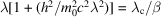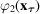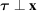International Tables for Crystallography (2010). Vol. B. ch. 2.5, pp. 297-402   | 1 | 2 | https://doi.org/10.1107/97809553602060000767

## Contents

• 2.5. Electron diffraction and electron microscopy in structure determination  (pp. 297-402)
• 2.5.1. Foreword  (pp. 297-299) | html | pdf |
• 2.5.2. Electron diffraction and electron microscopy  (pp. 299-307) | html | pdf |
• 2.5.2.1. Introduction  (p. 299) | html | pdf |
• 2.5.2.2. The interactions of electrons with matter  (pp. 299-301) | html | pdf |
• 2.5.2.3. Recommended sign conventions  (p. 301) | html | pdf |
• 2.5.2.4. Scattering of electrons by crystals; approximations  (pp. 301-302) | html | pdf |
• 2.5.2.5. Kinematical diffraction formulae  (pp. 302-303) | html | pdf |
• 2.5.2.6. Imaging with electrons  (pp. 303-304) | html | pdf |
• 2.5.2.7. Imaging of very thin and weakly scattering objects  (pp. 304-306) | html | pdf |
• 2.5.2.8. Crystal structure imaging  (p. 306) | html | pdf |
• 2.5.2.9. Image resolution  (pp. 306-307) | html | pdf |
• 2.5.2.10. Electron diffraction in electron microscopes  (p. 307) | html | pdf |
• 2.5.3. Point-group and space-group determination by convergent-beam electron diffraction  (pp. 307-356) | html | pdf |
• 2.5.3.1. Introduction  (pp. 307-308) | html | pdf |
• 2.5.3.2. Point-group determination  (pp. 308-318) | html | pdf |
• 2.5.3.2.1. Symmetry elements of a specimen and diffraction groups  (pp. 308-309) | html | pdf |
• 2.5.3.2.2. Identification of three-dimensional symmetry elements  (pp. 309-310) | html | pdf |
• 2.5.3.2.3. Identification of two-dimensional symmetry elements  (p. 310) | html | pdf |
• 2.5.3.2.4. Diffraction-group determination  (pp. 310-311) | html | pdf |
• 2.5.3.2.5. Point-group determination  (p. 311) | html | pdf |
• 2.5.3.2.6. Projection diffraction groups  (pp. 311-312) | html | pdf |
• 2.5.3.2.7. Symmetrical many-beam method  (pp. 312-318) | html | pdf |
• 2.5.3.3. Space-group determination  (pp. 318-344) | html | pdf |
• 2.5.3.3.1. Lattice-type determination  (p. 318) | html | pdf |
• 2.5.3.3.2. Identification of screw axes and glide planes  (pp. 318-323) | html | pdf |
• 2.5.3.3.3. Space-group determination  (pp. 323-333) | html | pdf |
• 2.5.3.3.4. Dynamical extinction in HOLZ reflections  (pp. 333-334) | html | pdf |
• 2.5.3.3.5. Symmetry elements observed by CBED  (p. 334) | html | pdf |
• 2.5.3.3.6. Examples of space-group determination  (pp. 334-344) | html | pdf |
• 2.5.3.4. Symmetry determination of incommensurate crystals  (pp. 344-352) | html | pdf |
• 2.5.3.4.1. General remarks  (pp. 344-348) | html | pdf |
• 2.5.3.4.2. Point-group determination  (pp. 348-349) | html | pdf |
• 2.5.3.4.3. Space-group determination  (pp. 349-352) | html | pdf |
• 2.5.3.5. Symmetry determination of quasicrystals  (pp. 352-356) | html | pdf |
• 2.5.3.5.1. Icosahedral quasicrystals  (pp. 352-354) | html | pdf |
• 2.5.3.5.2. Decagonal quasicrystals  (pp. 354-356) | html | pdf |
• 2.5.4. Electron-diffraction structure analysis (EDSA)  (pp. 356-361) | html | pdf |
• 2.5.4.1. Introduction  (p. 356) | html | pdf |
• 2.5.4.2. The geometry of ED patterns  (pp. 356-358) | html | pdf |
• 2.5.4.3. Intensities of diffraction beams  (pp. 358-359) | html | pdf |
• 2.5.4.4. Structure analysis  (pp. 359-361) | html | pdf |
• 2.5.5. Image reconstruction  (pp. 361-366) | html | pdf |
• 2.5.5.1. Introduction  (p. 361) | html | pdf |
• 2.5.5.2. Thin weak phase objects at optimal defocus  (pp. 361-362) | html | pdf |
• 2.5.5.3. An account of absorption  (pp. 362-363) | html | pdf |
• 2.5.5.4. Thick crystals  (p. 363) | html | pdf |
• 2.5.5.5. Image enhancement  (pp. 363-366) | html | pdf |
• 2.5.6. Three-dimensional reconstruction  (pp. 366-375) | html | pdf |
• 2.5.6.1. The object and its projection  (pp. 366-367) | html | pdf |
• 2.5.6.2. 3D reconstruction in the general case  (pp. 367-368) | html | pdf |
• 2.5.6.3. Discretization and interpolation  (pp. 368-369) | html | pdf |
• 2.5.6.4. The algebraic and iterative methods  (pp. 369-371) | html | pdf |
• 2.5.6.5. Filtered backprojection  (pp. 371-372) | html | pdf |
• 2.5.6.6. Direct Fourier inversion  (pp. 372-375) | html | pdf |
• 2.5.6.7. 3D reconstruction of symmetric objects  (p. 375) | html | pdf |
• 2.5.7. Single-particle reconstruction  (pp. 375-388) | html | pdf |
• 2.5.7.1. Formation of projection images in single-particle reconstruction  (pp. 375-376) | html | pdf |
• 2.5.7.2. Structure determination in single-particle reconstruction  (pp. 376-377) | html | pdf |
• 2.5.7.3. Electron microscopy and data digitization  (pp. 377-378) | html | pdf |
• 2.5.7.4. Assessment of the data quality and estimation of the image formation parameters  (p. 378) | html | pdf |
• 2.5.7.5. 2D data analysis – particle picking  (p. 379) | html | pdf |
• 2.5.7.6. 2D alignment of EM images  (pp. 379-381) | html | pdf |
• 2.5.7.7. Initial determination of 3D structure using tilt experiments  (pp. 381-382) | html | pdf |
• 2.5.7.8. Ab initio 3D structure determination using computational methods  (pp. 382-383) | html | pdf |
• 2.5.7.9. Refinement of a 3D structure  (pp. 383-385) | html | pdf |
• 2.5.7.10. Resolution estimation and analysis of errors in single-particle reconstruction  (pp. 385-386) | html | pdf |
• 2.5.7.11. Analysis of 3D cryo-EM maps  (pp. 386-388) | html | pdf |
• 2.5.8. Direct phase determination in electron crystallography  (pp. 388-394) | html | pdf |
• 2.5.8.1. Problems with `traditional' phasing techniques  (pp. 388-389) | html | pdf |
• 2.5.8.2. Direct phase determination from electron micrographs  (p. 389) | html | pdf |
• 2.5.8.3. Probabilistic estimate of phase invariant sums  (pp. 389-391) | html | pdf |
• 2.5.8.4. The tangent formula  (pp. 391-392) | html | pdf |
• 2.5.8.5. Density modification  (p. 392) | html | pdf |
• 2.5.8.6. Convolution techniques  (p. 392) | html | pdf |
• 2.5.8.7. Maximum entropy and likelihood  (pp. 392-393) | html | pdf |
• 2.5.8.8. Influence of multiple scattering on direct electron-crystallographic structure analysis  (pp. 393-394) | html | pdf |
• References | html | pdf |
• Figures
• Fig. 2.5.2.1. The variation with accelerating voltage of electrons of ( a ) the wavelength, λ and ( b ) the quantity(p. 301) | html | pdf |
• Fig. 2.5.2.2. Diagram representing the critical components of a conventional transmission electron microscope (TEM) and a scanning transmission electron microscope (STEM)  (p. 304) | html | pdf |
• Fig. 2.5.2.3. The functions, the phase factor for the transfer function of a lens given by equation (2.5.2.33), andfor the Scherzer optimum defocus condition  (p. 305) | html | pdf |
• Fig. 2.5.3.1. Four symmetry elements m ′, i , 2′ andof an infinitely extended parallel-sided specimen  (p. 309) | html | pdf |
• Fig. 2.5.3.2. Illustration of symmetries appearing in dark-field patterns (DPs)  (p. 310) | html | pdf |
• Fig. 2.5.3.3. Illustration of symmetries appearing in dark-field patterns (DPs) and a pair of dark-field patterns (±DP) for the combinations of symmetry elements  (p. 310) | html | pdf |
• Fig. 2.5.3.4. Relation between diffraction groups and crystal point groups  (p. 312) | html | pdf |
• Fig. 2.5.3.5. CBED patterns of Si taken with the  incidence  (p. 314) | html | pdf |
• Fig. 2.5.3.6. CBED pattern of Si taken with the  incidence  (p. 314) | html | pdf |
• Fig. 2.5.3.7. Illustration of symmetries appearing in hexagonal six-beam, square four-beam and rectangular four-beam dark-field patterns expected for all the diffraction groups except for 1, 1 R , 2, 2 R and 21 R   (pp. 315-317) | html | pdf |
• Fig. 2.5.3.8. CBED patterns of FeS 2 taken with the  incidence  (p. 320) | html | pdf |
• Fig. 2.5.3.9. CBED patterns of V 3 Si taken with the  incidence  (p. 321) | html | pdf |
• Fig. 2.5.3.10. Illustration of the production of dynamical extinction lines in kinematically forbidden reflections due to a b -glide plane and a 2 1 screw axis  (p. 322) | html | pdf |
• Fig. 2.5.3.11. CBED patterns obtained from ( a ) thin and ( b ) thick areas of (001) FeS 2   (p. 322) | html | pdf |
• Fig. 2.5.3.12. CBED pattern of FeS 2 taken with the  electron-beam incidence  (p. 322) | html | pdf |
• Fig. 2.5.3.13. Illustration of dynamical extinction lines appearing in HOLZ reflections due to glide planes  (p. 344) | html | pdf |
• Fig. 2.5.3.14. HOLZ CBED pattern of FeS 2   (p. 344) | html | pdf |
• Fig. 2.5.3.15. CBED patterns of rutile  (p. 345) | html | pdf |
• Fig. 2.5.3.16. CBED patterns of Sm 3 Se 4   (p. 346) | html | pdf |
• Fig. 2.5.3.17. The (3 + 1)-dimensional description of one-dimensionally modulated crystals  (p. 347) | html | pdf |
• Fig. 2.5.3.18. ( a ) Schematic diffraction pattern from a modulated crystal  (p. 347) | html | pdf |
• Fig. 2.5.3.19. CBED patterns of the incommensurate phase of Sr 2 Nb 2 O 7 taken at 60 kV  (p. 349) | html | pdf |
• Fig. 2.5.3.20. ( a ) Mirror symmetry of modulation waves () τ 4 = 0  (p. 350) | html | pdf |
• Fig. 2.5.3.21. ( a ) Umweganregung paths a , b and c to the 0011 forbidden reflection  (p. 350) | html | pdf |
• Fig. 2.5.3.22. Schematic diffraction pattern at the  incidence of Sr 2 Nb 2 O 7   (p. 351) | html | pdf |
• Fig. 2.5.3.23. Diffraction pattern of Sr 2 Nb 2 O 7 taken with  incidence at 60 kV  (p. 351) | html | pdf |
• Fig. 2.5.3.24. Three pairs of ZOLZ [( a ), ( c ) and ( e )] and HOLZ [( b ), ( d ) and ( f )] CBED patterns taken at 60 kV from an area of Al 74 Mn 20 Si 6   (p. 352) | html | pdf |
• Fig. 2.5.3.25. CBED patterns of Al 74 Mn 20 Si 6 taken with an electron incidence along the threefold axis  (p. 353) | html | pdf |
• Fig. 2.5.3.26. CBED patterns of metastable Al 70 Ni 15 Fe 15 taken from a 3 nm diameter area  (p. 353) | html | pdf |
• Fig. 2.5.3.27. CBED patterns of metastable Al 70 Ni 20 Fe 10 taken from a 3 nm diameter area  (p. 355) | html | pdf |
• Fig. 2.5.4.1. Ewald spheres in reciprocal space  (p. 357) | html | pdf |
• Fig. 2.5.4.2. Triclinic reciprocal lattice  (p. 357) | html | pdf |
• Fig. 2.5.4.3. Formation of ellipses on an electron-diffraction pattern from an oblique texture  (p. 358) | html | pdf |
• Fig. 2.5.5.1. The χ function and two components of the Scherzer phase function sinand cos(p. 362) | html | pdf |
• Fig. 2.5.5.2. ( a ) Diagram of an optical diffractometer  (p. 365) | html | pdf |
• Fig. 2.5.6.1. A three-dimensional objectand its two-dimensional projection(p. 367) | html | pdf |
• Fig. 2.5.6.2. The projection sphere and projectionofalong τ onto the plane(p. 367) | html | pdf |
• Fig. 2.5.6.3. ( a ) Formation of a backprojection function; ( b ) projection synthesis (2.5.6.9) is a superposition of these functions  (p. 368) | html | pdf |
• Fig. 2.5.6.4. Discretization in two dimensions ( d = 2)  (p. 368) | html | pdf |
• Fig. 2.5.6.5. Nonuniform distribution of projections  (p. 371) | html | pdf |
• Fig. 2.5.6.6. Kaiser–Bessel window function used in the gridding reconstruction algorithm GDFR  (p. 373) | html | pdf |
• Fig. 2.5.6.7. Partitions of the sampling Fourier space using Voronoi diagrams in direct inversion algorithms  (p. 374) | html | pdf |
• Fig. 2.5.6.8. ( a ) Plot of correlation coefficients (CCs) calculated between Fourier transforms of the reconstructed structure and the original phantom as a function of the magnitude of the spatial frequency using five reconstruction algorithms  (p. 374) | html | pdf |
• Fig. 2.5.7.1. Typical steps performed in a single-particle cryo-EM structure determination project  (p. 377) | html | pdf |
• Fig. 2.5.7.2. The geometrical constraints of the 2D alignment problem  (p. 380) | html | pdf |
• Fig. 2.5.7.3. Principle of random conical tilt reconstruction  (p. 381) | html | pdf |
• Fig. 2.5.7.4. Schematic of the 3D projection-alignment procedure  (p. 384) | html | pdf |
• Fig. 2.5.7.5. Schematic of 3D projection alignment with CTF correction performed on the level of 3D maps reconstructed from projection images sorted into groups that share similar defocus settings  (p. 384) | html | pdf |
• Fig. 2.5.7.6. A high-resolution cryo-EM map allows backbone tracing  (p. 387) | html | pdf |
• Fig. 2.5.7.7. Hrs (blue) embedded into the membrane (yellow) of an early endosome  (p. 387) | html | pdf |
• Fig. 2.5.8.1. Potential map for diketopiperazine  (p. 390) | html | pdf |
• Tables
• Table 2.5.2.1. Standard crystallographic and alternative crystallographic sign conventions for electron diffraction  (p. 302) | html | pdf |
• Table 2.5.3.1. Two- and three-dimensional symmetry elements of an infinitely extended parallel-sided specimen  (p. 309) | html | pdf |
• Table 2.5.3.2. Symmetry elements of an infinitely extended parallel-sided specimen and diffraction groups  (p. 309) | html | pdf |
• Table 2.5.3.3. Symmetries of different patterns for diffraction and projection diffraction groups  (p. 311) | html | pdf |
• Table 2.5.3.4. Diffraction groups expected at various crystal orientations for 32 point groups  (pp. 313-314) | html | pdf |
• Table 2.5.3.5. Symmetries of hexagonal six-beam CBED patterns for diffraction groups  (p. 318) | html | pdf |
• Table 2.5.3.6. Symmetries of square four-beam CBED patterns for diffraction groups  (p. 319) | html | pdf |
• Table 2.5.3.7. Symmetries of rectangular four-beam CBED patterns for diffraction groups  (p. 319) | html | pdf |
• Table 2.5.3.8. Dynamical extinction rules for an infinitely extended parallel-sided specimen   (p. 323) | html | pdf |
• Table 2.5.3.9. Dynamical extinction lines appearing in ZOLZ reflections for all crystal space groups except Nos. 1 and 2  (pp. 324-332) | html | pdf |
• Table 2.5.3.10. Space-group sets indistinguishable by dynamical extinction lines  (p. 333) | html | pdf |
• Table 2.5.3.11. Space-group sets distinguishable by coherent CBED  (p. 333) | html | pdf |
• Table 2.5.3.12. Dynamical extinction lines appearing in HOLZ reflections for crystal space groups that have mirror and glide planes  (pp. 335-343) | html | pdf |
• Table 2.5.3.13. Wavevectors, point- and space-group symbols and CBED symmetries of one-dimensionally modulated crystals  (p. 349) | html | pdf |
• Table 2.5.3.14. Diffraction groups and CBED symmetries for two icosahedral point groups  (p. 353) | html | pdf |
• Table 2.5.3.15. Pentagonal and decagonal point groups constructed by analogy with trigonal and hexagonal point groups  (p. 354) | html | pdf |